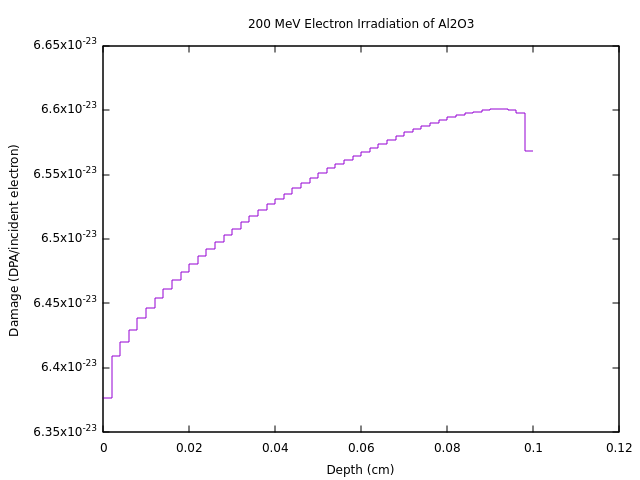Good day, I am trying to produce radiation damage plots similar to figure 6 of this paper:

xMeV_Electrons_Al203_dpa.flair (2.0 KB)
xMeV_Electrons_Al203_dpa.inp (2.6 KB)

As can be seen in the input card I have provided this is as far as I have managed to come however the output from running this is vastly different to that seen in the paper mentioned above.

In this simulation I wish to simulate the radiation damage induced by an Electron Beam of some MeV energies onto a Al2O3 sample with the defined geometry. If anyone is able to help me better understand the disparity between my results and those in the paper I would be extremely grateful.

Many thanks
Cameron Baldwin

Dear @cbaldwin,

Would you care to elaborate what you mean when saying that there results are vastly different?
The figure you’re referring to shows the DPA for a Ge ion beam, while you’re Fluka input simulates an electron beam. This is already quite a good reason to explain a lot of differences.

Hi @amario ,

I understand the differences that would occur, however the magnitude of the differences is what I don’t understand. When I plot the results from my simulations they look like this (see attached). Thank you for your reply.Figure 6 results appear to be normalized to 10^20 beam particles or so (see Table 2), while your plot gives the DPA per one electron.

Hi @ceruttif,

Are you sure this is the case? Where then in my input can I make a change to give the total DPA?

I’m sure, in view of the expected order of magnitude for one single incident particle, which is what you get (the remaining discrepancy is due to the different beam type, as @amario pointed out).
Nowhere in your input you shall normalize to your beam intensity/current. As for any FLUKA result (apart from activation results that are already normalized to the input irradiation profile), this kind of normalization has to be done at post-processing level, for instance in the Flair Plot frame generating your plot, where there is a dedicated Norm field.

Hi @ceruttif,

Thank you very much. I am beginning to understand. One thing I now do not understand is when you say that figure 6 has been normalized to 10^20 events, I see where to do this in the Norm field in the plotting section. How is this norm different from the start card where you specify the number of primaries (events). I thought that this number was the total number of particles one wished to simulate?

Dear @cbaldwin,

Fluka results are normalized per primary particles, have a look at the notes in the USRBIN card manual..
Then you would have to multiply this results to the number of particles in your impulse to compare to the plot in the paper.

Hi @amario

I’m not sure I understand still. If I wish to simulate, for argument’s sake 10^20 total electrons interacting with my sample. If I set the primaries to for example 10^5 would I have to normalize post-processing to 10^15?

I apologize if I’ll appear pedant, but I think there are two concepts that need to be clarified.

1-No matter how many primary one simulates, Fluka results will always be given per single primary (this is not exactly true when using biasing or considering induced activation, but let’s not be too much pedant).
No matter whether you simulate 100 primaries or 100 billions primaries, Fluka will tell you how much energy is deposited in your target by one single primary. The only difference that follows from using a given number of primaries is the statistical uncertainty that comes with your estimate.

2-Fluka results then need to be normalized to a quantity that make sense for the specific study case.
If you want to know how much energy is deposited by a single bunch accelerated in your machine, then you have to multiply the Fluka result per primary for the number of primaries that are in your bunch which could be whatever 10^9, 10^10, or even, provided that you perform a proper conversion 1nA.

Getting to your specific case, if you want to know how much energy (or DPA, or dose, or whatever quantity) is deposited by one bunch that contains 10^20 particles, then you will have to multiply the Fluka results (given per single primary) time 10^20, regardless of the number of primaries you have simulated.

I hope it is more clear now and I apologize if I sounded patronizing.

Thank you very much I really appreciate the help.

Many thanks